# Themes, topics - math word problems

#### Number of problems found: 1081

• ShortcutThe road from the cottage to the shop 6 km away leads either along a straight road that the bicycle can drive at a speed of 18 km/h or by "shortcut". It measures only 3.6 km. But the road from the cottage is all uphill - at a speed of 8 km/h, you can go h
• Average speed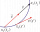The car drove on one section of the highway for half an hour at a speed of 80 km/h. Then he reduced his speed to 60km/h. It went three-quarters of an hour at a speed of 60 km/h. What was the average speed of the car?
• Resistor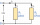Resistor 1 with a resistance of 100 Ohms and resistor 2 with a resistance of 400 Ohms are connected side by side in the circuit. There is a voltage of 80V between the resistor terminals. a) Draw a circuit diagram and write the entered quantities in it b)
• Three resistors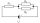You have to connect three identical resistors with 12 Ohm resistances so that you gradually get the resulting resistance a) 36 Ohm, b) 4 Ohm, 18 Ohm. Draw a diagram of each circuit.
• Three days way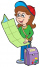The tourist walked a quarter of the way on the first day, a third of the rest on the second day and 20 km on the last day. How many km did he cover in three days?
• A cheetah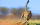A cheetah was separated from his brothers on a hunting mission.  The brothers were at the watering hole 120 km away.  The lost cheetah knew that he had to make it back before sundown which was 1.5 hours away.  His brothers had planned to leave the waterin
• Two carsTwo cars (trucks and passenger) drove simultaneously from two places 50 km away. If they go against each other, they will meet in 30 minutes. If they follow each other in the same direction, the car will catch up with the truck in 2.5 hours. What is the s
• Thermometer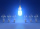Immerse a thermometer with a heat capacity of 2.0 J. K-1 in water weighing 67.0 grams. Before immersion in water, the thermometer showed a temperature of 17.8 degrees Celsius. After reaching equilibrium, the temperature is 32.4 degrees. What was the water
• Six identical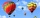Six identical compressors inflate 180 balloons in one hour. How many balloons inflate one compressor in 10 minutes?
• ThereThere is 5 l of water with a temperature of 20°C in the bucket. We add 3 liters of hot water. The resulting temperature will be 30°C. What was the temperature of the hot water?
• Frequency of oscillation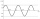A weight of 1 kg was suspended from the spring and the spring was extended by 1.5 cm. Determine the frequency of the natural oscillation of the resulting oscillator.
• Two pendulums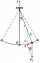In 24 s, the first pendulum performed 12 oscillations and the second 16 oscillations. Determine the periods and frequencies of both pendulums.
• Part-timers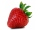Six part-timers would take seven hours to pick strawberries. How long would this work take if two more temporary workers were added after three hours?
• What power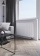What power should the radiator have in a room 5x4 meters with a height of 3 meters? Hint: Calculate 35 watts per meter ^ 3 in case you need 22 degrees in the room but you need about 24 degrees in the bathroom. It's an old heating cheat, but it will defini
• Two workers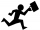One worker makes a part 4 hours and the second 9 hours later than they would make the same part together. How long does it take for each worker to make the part himself?
• Above water surface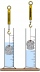If we remove the stone from the water, we apply a force of 120N. How much force will we have to exert if we move the stone above the water surface? The density of the stone is 5000 kg/m ^ 3.
• Dynamometer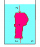What is the volume of a body that stretches a dynamometer in air, on which it is suspended by a force of 2.5 N, and if it is immersed in alcohol with a density of 800 kg/m ^ 3, does it tension the dynamometer with a force of 1.3 N?
• Draw a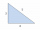Draw a triangle ABC, if you know: alpha = 60° side b = 4 cm side a = 10 cm
• Bike pathBill was riding his bike on a bike path that was 5 1/4 miles long. He rode his bike awhile and stopped for s drink or water. Then he rode 2 7/10 miles and was at the end of the path. How far had Bill ridden before he stopped for water?
• Direction indicatorAs that which the electrical voltage is connected to the lamp direction indicator 7400 mOhm resistance when current of 1.6 A passes through it?

Do you have an interesting mathematical word problem that you can't solve it? Submit a math problem, and we can try to solve it.

We will send a solution to your e-mail address. Solved examples are also published here. Please enter the e-mail correctly and check whether you don't have a full mailbox.

Please do not submit problems from current active competitions such as Mathematical Olympiad, correspondence seminars etc...